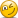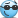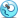GARP.FRM.PQ.P22016 GARP PQ - Question 5 - CDS (garp16-p2-5)

no_ming

Member
Hi, Mr. Harper, I would like to know whether the following 2 question (Valuation of CDS & Sklar's theorem) are still in the syllabus as I cannot find the formula in the notes. ThanksLast edited:

David Harper CFA FRM

David Harper CFA FRM
Staff member
Subscriber
Hi @no_ming Yes, both are clearly in the syllabus. CDS valuation never left the syllabus (in 10+ years) although previously it was assigned to Hull's chapter on Credit derivatives. Thanks,

Arnaudc

Member
Hi all,
This is my first post on this forum (which I regularly consult for all the valuable information's in it).
On question 5, I fail to understand why couldn't we simply use the formula:
PD = Hazard Rate = Spread / (1-R). Using this would give a Spread of exactly 560 bps.

Could someone tell me what is wrong in my rationale?
Thank you very much.

Kind regards,

David Harper CFA FRM

David Harper CFA FRM
Staff member
Subscriber
Hi @Arnaudc Welcome to the forum! This CDS question is essentially similar to https://forum.bionicturtle.com/threads/2013-garp-practice-exam-p2-question-6.7039/
As with that problem, this approximation would work except the CDS has a mechanical feature difference from the bond, under the assumptions given at least. Specifically, from what I can tell the following typical assumption explains almost the entire different between your approximation and the more exact answer given: "Assuming defaults can only occur halfway through the year and that the accrued premium is paid immediately after a default."

To see why, imagine there was no accrual paid upon default. Then the solution would be given by:
Premium leg = [0.5*0%*d(0.5)] + [100%*d(1.0)] and Payoff leg = same = 80% * 7% * d(0.5), such that
0.975895*S = 0.05465, and S = 560.1 (versus your 560 approximation!).

To recap,
• The CDS assumes: Premium leg = [0.5 * 7% default * d(0.5)] + [93% survival *d(1.0)]
I hope that explains, thanks!

Last edited:

Arnaudc

Member
@David Harper CFA FRM ,
Thank you very much for your time answering my question. You made a point by stressing the importance of assumptions to assess which models could/should be used.

I still have a point needed to be clarified.
The reason why you assume 100% of premium payment seems related to the fact that you are certain you would receive the premium at the end of 1 year? Is it because default or non default, in any cases, the premium is paid at the end of the year 1?
In this case I understand and a higher spread is intuitive as a default half year means we would receive only half of the premium we were promised.

EDIT:
Actually the "simplified formula" is based on the assumption that the spread should equal your EL% (PD x LGD). In my case, 7% x 80% = 5.60%. Absent any convention of discounting / in between period default, the spread should be equal to EL% (as it equals paying leg with contingent leg)

Again, thank you for your time, I really appreciate what you are doing for the community of learners we are.

Last edited:

David Harper CFA FRM

David Harper CFA FRM
Staff member
Subscriber
Hi @Arnaudc Yes, exactly, I agree with your edit. My re-casting of the CDS valuation equality (which is to solve for spread by assuming the PV of the expected premiums paid must equal the PV of the contingent payoff, both probability-adjusted) is simply to re-cast into the approximation that you used. In other words,
• pv[Premium leg] = pv[Contingent Payoff] is technically exact, and probability-adjusted translates in this case into
• pv[7% prob of 0.5 accrued premium + 93% prob of full premium] = pv[7% prob of 80% payoff]; but, if we just "eliminate the unique CDS feature" and assume the premium is paid regardless then
• pv[100% prob of full premium] = pv[7% prob of 80% payoff], which is, just as you say, equivalent to spread = EL = LGD*PD. A potential confusion, for me at least, is the 80%: is the 80% recovery or LGD? It is the CDS recovery but LGD to the protection seller, so i think it's easy to select the wrong 20%/80% here. But we're pricing the CDS, and so the spread is compensation for the 80% that will be paid by the protection seller. Thank for helping me think it through!

Arnaudc

Member
@David Harper CFA FRM ,
Thank you for confirming / clarifying!
Regarding the LGD confusion, I think an easy way not to get it wrong is to understand the LGD as the loss an investor would suffer an a specific instrument without taking into account CDS protection. (then this LGD is simply plugged into CDS valuation formula's.

Thanks again.

Kind regards,

bpdulog

Active Member
Hi all,

How would this #5 problem work if this were extended to 1.5 years? Or 2 years?

In addition, I couldn't really find a related example in the text - is this the Merrill Lynch CDS curve example in Malz 7?

bpdulog

Active Member
The formula posted above is the same as in the problem solution, but what does the 1st term represent? And how do you extend this past 1 year?

Last edited:

David Harper CFA FRM

David Harper CFA FRM
Staff member
Subscriber
Hi @bpdulog Malz explains his formula but it's essentially identical the (probably easier to follow) CDS valuation in Hull Chapter 25, and as discussed above, GARP's solution conforms (IMO) except, as you note, it's just a "truncated" example where the maturity is only one year. Below I input the same assumptions into a 5-year CDS. Although the dynamics (including discounting) imply that the spread shouldn't vary much with the maturityWith respect to the Malz formula (above), his difference is that he assumes the spread is paid in quarterly installments such that if the spread is expressed in basis points, each quarterly installment is *1/4 and to retrieve the decimal from, say 150 bps, we divided by 10,000 (note familiarity with DV01) such that 200 bps = 200/10,000 = 200/(10^4) = 0.02; hence the 1/(4*10^4). Here is the XLS https://www.dropbox.com/s/wq4ple3wgb0j387/0420-cds-valuation.xlsx?dl=0 for the valuation below (which extends 2016 Q5 above to five years with the same assumptions). Thanks,bpdulog

Active Member
Hi @bpdulog Malz explains his formula but it's essentially identical the (probably easier to follow) CDS valuation in Hull Chapter 25, and as discussed above, GARP's solution conforms (IMO) except, as you note, it's just a "truncated" example where the maturity is only one year. Below I input the same assumptions into a 5-year CDS. Although the dynamics (including discounting) imply that the spread shouldn't vary much with the maturityWith respect to the Malz formula (above), his difference is that he assumes the spread is paid in quarterly installments such that if the spread is expressed in basis points, each quarterly installment is *1/4 and to retrieve the decimal from, say 150 bps, we divided by 10,000 (note familiarity with DV01) such that 200 bps = 200/10,000 = 200/(10^4) = 0.02; hence the 1/(4*10^4). Here is the XLS https://www.dropbox.com/s/wq4ple3wgb0j387/0420-cds-valuation.xlsx?dl=0 for the valuation below (which extends 2016 Q5 above to five years with the same assumptions). Thanks,Huge thank you for this!!!! It is interesting to see there's no pricing change for extended periods, due to the constant hazard rate assumption I suppose. It's also cool to play around with the inputs and see how much the spread goes up and down

David Harper CFA FRM

David Harper CFA FRM
Staff member
Subscriber
@bpdulog Sure thing! Although I've revised all of Hull P1, I haven't gotten to the Hull P2 XLS revisions yet. I agree about the (lack of) a pricing change. I tested at several different maturities and I wish I had more time to explore the exact reason why there is basically no change. My expectation actually (was) to see an inflation trend; e.g., extending adds a cost, sort of like a term premium for protection. So this outcome surprises me. I worry it's wrong, however the underlying variables are changing, appropriately, and I can't find any mechanical issues in the extension. I'm just surprised that under this method, the spread seems to be basically invariant to the CDS maturity. It doesn't feel correct b/c it feels like the protection buyer should experience an upward drift (although this sort of "term risk premium" admittedly isn't in the model). Hmmm ... I not satisfied with my own level of intuitive understanding hereeg, when I have time, i want to see if i can "break" this invariance with changing assumption(s) ... but I am glad you asked the question!

Srilakshmi

Member
can someone please qn 5- the calculation of the CDS spread. Thanks!

Last edited by a moderator:

Nicole Seaman

Director of FRM Operations
Staff member
Subscriber
can someone please qn 5- the calculation of the CDS spread. Thanks!

@Srilakshmi

Thank you,

Nicole

Staff member
Subscriber

Srilakshmi

Member
Thank you David and Nicole! Appreciate it.

RushilChulani

New Member
Hi @David Harper CFA FRM, thank you for the uploading the excel file though I fail to understand the Expected Payments part completely in the 5 year situation. How I read the question is that the Expected Payments over 5 years is [(PV of prob of survival in year 1 + PV of Prob of Survival over 2 years + Prob of Survival over 3 years + .......) * s] + [(PV of prob of default in 1 year + PV of prob of default in 2 years + ...) * s]. What I am trying to get to is that in the Prob of survival part, aren't we double-counting the previous years each time we add the PV of an extended year, so for the 5 years we have considered the prob of survival through 5 years and not just the prob of survival in year 5, which means we include the possibility of default in year 1. Since, we already consider the prob of survival in year 1 as the first term, I feel like there is double-counting. Where am I going wrong?

Thanks,

Rushil

Hi @bpdulog Malz explains his formula but it's essentially identical the (probably easier to follow) CDS valuation in Hull Chapter 25, and as discussed above, GARP's solution conforms (IMO) except, as you note, it's just a "truncated" example where the maturity is only one year. Below I input the same assumptions into a 5-year CDS. Although the dynamics (including discounting) imply that the spread shouldn't vary much with the maturityWith respect to the Malz formula (above), his difference is that he assumes the spread is paid in quarterly installments such that if the spread is expressed in basis points, each quarterly installment is *1/4 and to retrieve the decimal from, say 150 bps, we divided by 10,000 (note familiarity with DV01) such that 200 bps = 200/10,000 = 200/(10^4) = 0.02; hence the 1/(4*10^4). Here is the XLS https://www.dropbox.com/s/wq4ple3wgb0j387/0420-cds-valuation.xlsx?dl=0 for the valuation below (which extends 2016 Q5 above to five years with the same assumptions). Thanks,David Harper CFA FRM

David Harper CFA FRM
Staff member
Subscriber
Hi @RushilChulani I think I do understand why that's not obviously intuitive. After all, it's different than how the contingent payoff is treated which may be more intuitive in contrast. After all, the contingent payoff probabilities are unconditional (aka, joint) default probabilities which means they are mutually exclusive and naturally additive; for example, the year 5 payoff pd is only 1.84% because it is a joint probability given by 92.2% cumulative probability thru the end of year 4 (the prior year) * 2.0% conditional probability. On this payoff side, perhaps it is more intuitive because the 2.0% is a conditional PD such that we don't perceive the double-counting.

But the difference on the payment side is that the protection buyer pays the spread every year as long as the reference survives (as opposed to the protection seller who only pays once, if at all); i.e., if the reference never defaults, there will be five (not one!) spread payments. If there were only one spread payment at the end of five years, we would be double-counting. But in this case of a "stream of spreads" we are merely weighting the stream of payments by their probability of occurring. Let A(t) be the annuity factor; i.e., the sum of discount factors, which is 4.317. Imagine that survival is guaranteed, in which case five (5) spreads are paid with a present value = 4.317*S. Implicitly that would be to assign 100% to each of the five cumulative probabilities because each spread is guaranteed: S*100%*0.951 + S*100%*0.905 ... S*100%*0.729. Notice how that isn't double-counting? So these probabilities are just weighting each year's spread payment. I hope that's helpful!

RushilChulani

New Member
Hi @David Harper CFA FRM, Thank you for responding! I yet am bothered by the fact that we don't consider the survival for just the specific year. So for example, why isn't it 0.9802 * 0.9512 for year 1, [1-(0.9802-9608)] * 0.9048 for year 2, ...., [1-(0.9231-9048)] * 0.7788 for year 5. Won't that be the probability of survival with that specific year and not probability of survival since year 1?

(I used Hull's Ninth edition as reference and so the numbers are different. The idea is {1-[Prob of survival (n-1) - Prob of survival (n)]} for year "n")

Rushil

Hi @RushilChulani I think I do understand why that's not obviously intuitive. After all, it's different than how the contingent payoff is treated which may be more intuitive in contrast. After all, the contingent payoff probabilities are unconditional (aka, joint) default probabilities which means they are mutually exclusive and naturally additive; for example, the year 5 payoff pd is only 1.84% because it is a joint probability given by 92.2% cumulative probability thru the end of year 4 (the prior year) * 2.0% conditional probability. On this payoff side, perhaps it is more intuitive because the 2.0% is a conditional PD such that we don't perceive the double-counting.

But the difference on the payment side is that the protection buyer pays the spread every year as long as the reference survives (as opposed to the protection seller who only pays once, if at all); i.e., if the reference never defaults, there will be five (not one!) spread payments. If there were only one spread payment at the end of five years, we would be double-counting. But in this case of a "stream of spreads" we are merely weighting the stream of payments by their probability of occurring. Let A(t) be the annuity factor; i.e., the sum of discount factors, which is 4.317. Imagine that survival is guaranteed, in which case five (5) spreads are paid with a present value = 4.317*S. Implicitly that would be to assign 100% to each of the five cumulative probabilities because each spread is guaranteed: S*100%*0.951 + S*100%*0.905 ... S*100%*0.729. Notice how that isn't double-counting? So these probabilities are just weighting each year's spread payment. I hope that's helpful!

Last edited: